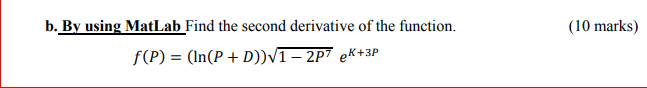### Create an Account

Already have account?

### Forgot Your Password ?

Home / Questions / (10 marks) b. By using MatLab Find the second derivative of the function. f(P) = (In(P + D...

# (10 marks) b. By using MatLab Find the second derivative of the function. f(P) = (In(P + D))1 - 2P7 eR+3P

(10 marks) b. By using MatLab Find the second derivative of the function. f(P) = (In(P + D))1 - 2P7 eR+3P

D = 1Apr 15 2021 View more View Less

#### Answer (Solved)Subscribe To Get Solution